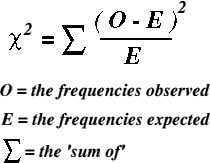# Statistical Methods – Chi-Square and 2×2 tables

Definition

When data is binary (i.e. exposure and outcome have only 2 options) the data can be plotted into a trusty 2×2 table (*they really are trusty – they pop up all over the place!)

The Chi-Square statistic (denoted x^2) is a non-parametric test which examines whether there is an association between 2 variables of a sample.  It determined if a distribution of observed frequencies differ from expected frequencies

• Measured variables much be independent
• Values of independent and dependent variables must be mutually exclusive
• Data must be raw numbers (e.g. nominal or ordinal)
• Data must be randomly drawn from the population
• Observed frequencies must not be too small (as expected No. must be >5… if <5 then Fisher’s Exact Test must be used)

Formulae, and how to…1. For each observed number, calculate the expected number = [(row total x col total) / table total]
2. Subtract expected from observed [O-E]
3. Square the result and divide by the expected number [(O-E)^2 / e]
4. Chi-Square = Sum of results for all cells

In 2×2 table the cut-off for 95% significance level (p = 0.05) = 3.84 (1.96^2).

If Chi-square is less than 3.84 we can say the result was statistically significant (at the 95% level)

The bigger the chi-square result, the more statisically significant it will be

DONE!

Advertisements